Page

# PYTHAGOREAN THEOREM

The Pythagorean Theorem states that, in a right triangle, the two smaller squares made from the triangles legs add up to equal the biggest square made from the hypotenuse.The lengths of the legs are commonly labeled as a and b and the hypotenuse length is labeled as c.REMEMBER:

HYPOTENUSE: c is the longest side of the triangle

LEGS: a and b are the other two sidesClick here  to find the missing hypotenuse , to find the missing leg, here  to find the perimeter, here  to do real world problems, and here to see if the triangle is a right triangle or not. You will use the Pythagorean Theorem online and get automatic feedback (it grades it)! 🙂

## What is the Pythagorean Theorem?

In short the Pythagorean Theorem can be explain with the formula below.

## a2 + b2 = c2

AGAIN, THIS IS ONLY TRUE FOR RIGHT TRIANGLES!

Here are other examples: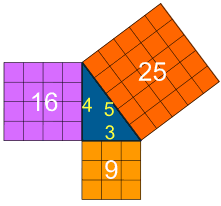Click here  to find the missing hypotenuse , here  to find the missing leg, here  to find the perimeter, here  to do real world problems, and here to see if the triangle is a right triangle or not. You will use the Pythagorean Theorem online and get automatic feedback (it grades it)! 🙂

# ANGLE and TRIANGLE RELATIONSHIPS

## Degrees

A degree is a unit of measurement used to measure angles.

### We can measure all Angles in Degrees.

There are 360 degrees in one Full Rotation (one
complete circle around

## The Degree Symbol: °

We use a little circle ° following the number to mean degrees.

For example 90° means 90 degrees

## Angles### In One Diagram

This diagram might make it easier to remember:

Also: Acute, Obtuse and Reflex are in alphabetical order.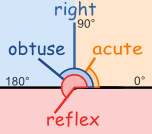# Supplementary Angles

Two Angles are Supplementary if they add up to 180 degrees.
 These two angles (140° and 40°) are Supplementary Angles, because they add up to 180°.Notice that together they make astraight angle.But the angles don’t have to be together.These two are supplementary because 60° + 120° = 180°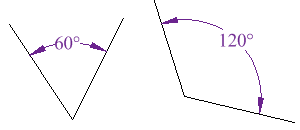# Complementary Angles

Two angles are Complementary when they
add up to 90 degrees
(a Right Angle).

 These two angles (40° and 50°) are Complementary Angles, because they add up to 90°.Notice that together they make a right angle.But the angles don’t have to be together.These two are complementary because 27° + 63° = 90°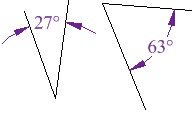# Angle Relationships

Click here, here, and here to find the missing angles of triangles and parallel lines cut by a transversal online and get automatic feedback (it grades it)! 🙂

# Interior Angle

### An Interior Angle is an angle inside a shape.# Exterior Angle

### The Exterior Angle is the angle between any side of a shape, and a line extended from the next side.# Angles On One Side of A Straight Line

### Angles on one side of a straight line will always add to 180 degrees.

If a line is split into 2 and you know one angle you can always find the other one.30° + 150° = 180°

# Interior Angles of Polygons

### An Interior Angle is an angle inside a shape.## Triangles

The Interior Angles of a Triangle add up to 180°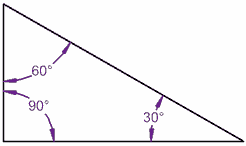### 80° + 70° + 30° = 180°

It works for this triangle!

Let’s tilt a line by 10° …

It still works, because one angle went up by 10°, but the other went down by 10°

## Quadrilaterals (Squares, etc)

(A Quadrilateral has 4 straight sides)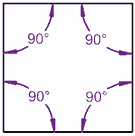### 80° + 100° + 90° + 90° = 360°

A Square adds up to 360°

Let’s tilt a line by 10° … still adds up to 360°!

The Interior Angles of a Quadrilateral add up to 360°

## Because there are Two Triangles in a Square

 The interior angles in this triangle add up to 180°(90°+45°+45°=180°)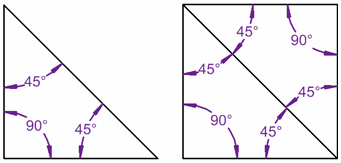Shape Term Definitionperpendicular line segments Two line segments which cross to form 90 degree angles.right angle A 90 degree angle.equilateral triangle A triangle with all sides equal and all angles equal.scalene triangle A triangle having three unequal sides and angles.vertex The intersection point of two sides of a plane figure.right triangle A triangle with one internal angle equal to 90 degrees.pentagon A polygon with 5 sides and 5 angles.square A rectangle having all four sides of equal length.intersecting line segments Line segments that cross each other.acute angle An angle less than 90 degrees but greater than 0 degrees.chord The line segment between two points on a given curve.radius A straight line extending from the center of a circle or sphere to the circumference or surface.line segment One part of a line.line A continuous extent of length.point A position in space.parallelogram A quadrilateral having both pairs of opposite sides parallel to each other.(Note: squares and rectangles are also quadrilaterals.)rectangle A parallelogram having four right angles.(Note: a square is also a rectangle.)rhombus An equilateral parallelogram having oblique angles.parallel line segments Line segments that do not intersect.quadrilateral A polygon with four sides.(Note: squares, rectangles and trapezoids are also quadrilaterals.)octagon A polygon having eight angles and eight sides.circle A closed plane curve consisting of all points at a given distance from a point within it called the center.trapezoid A quadrilateral plane figure having two parallel and two nonparallel sides.ray The part of a straight line considered as originating at a point on the line and as extending in one direction from that point.closed curve A curve that is continuous and has endpoints that meet at the same point.isosceles triangle A triangle which has two sides equal.hexagon A polygon having six sides and six angles.diameter A straight line passing through the center of a circle or sphere and meeting the circumference or surface at each end.obtuse angle An angle greater than 90 degrees but less than 180 degrees.